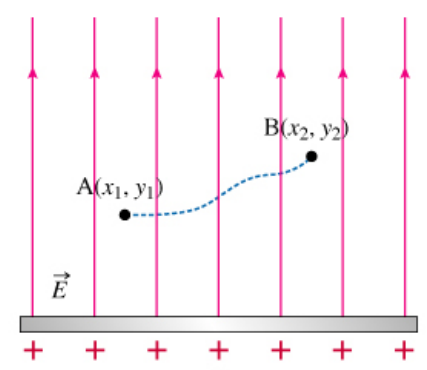# Problem: What is the potential difference VAB = VA − VB between points A and B?Express your answer in terms of some or all of E, x1, y1, x2, and y2.

###### FREE Expert Solution

The potential difference, ΔV:

$\overline{){\mathbf{∆}}{\mathbf{V}}{\mathbf{=}}{\mathbf{-}}\stackrel{\mathbf{⇀}}{\mathbf{E}}{\mathbf{·}}\stackrel{\mathbf{⇀}}{\mathbf{s}}}$

Vectors A and B are expressed as:

$\stackrel{\mathbf{⇀}}{\mathbf{A}}\mathbf{=}{\mathbit{x}}_{\mathbf{1}}\stackrel{\mathbf{^}}{\mathbf{i}}\mathbf{+}{\mathbit{y}}_{\mathbf{1}}\stackrel{\mathbf{^}}{\mathbf{j}}$

and

$\stackrel{\mathbf{⇀}}{\mathbf{B}}\mathbf{=}{\mathbit{x}}_{\mathbf{2}}\stackrel{\mathbf{^}}{\mathbf{i}}\mathbf{+}{\mathbit{y}}_{\mathbf{2}}\stackrel{\mathbf{^}}{\mathbf{j}}$

The displacement vector, s:

97% (458 ratings)###### Problem Details

What is the potential difference VAB = VA − VB between points A and B?

Express your answer in terms of some or all of E, x1, y1, x2, and y2.